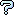All about flooble | fun stuff | Get a free chatterbox | Free JavaScript | Avatarsperplexus dot infoClassical Rules 1 (Posted on 2006-04-17)Find three positive rational numbers such that their sum is a square, and the sum of any pair exceeds the third by a square.

Classical Rules: Let a "square" be any number that is the square of a rational number.

 No Solution Yet Submitted by goFish Rating: 3.5000 (4 votes)Comments: ( Back to comment list | You must be logged in to post comments.)re: Full solution| Comment 5 of 10 |(In reply to Full solution by Federico Kereki)

But for x=4 and y=7, x^2 + y^2 = 65, not 63.

Also, a + b + c = 50/2 + 17/2 + 65/2 = 132/2 = 66, which I'm not sure is a square....

I got as far as the x^2 + y^2 + z^2 = t^2, but then I gave up...I seem to recall though that numbers that can be expressed as the sum of three squares all follow the same form (is it 4n+3 or something?).  Something like that.  Anyway, would it be possible to look for squares that also fit that form?

 Posted by tomarken on 2006-04-17 18:34:15Please log in:

 Search: Search body:
Forums (3)